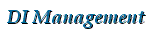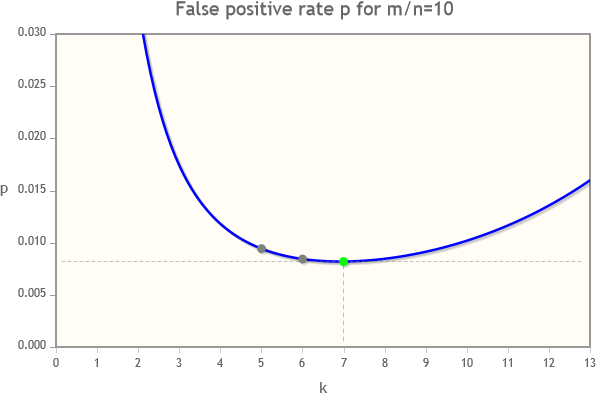DI Management Home > Mathematics > Algorithms > Bloom filters

#Bloom filters

This page looks at Bloom filters, a useful algorithm in computer science, and a Bloom filter calculator to find the optimum parameters.

## Background

A Bloom filter is an efficient technique to query if $x$ is a member of a set, $S$. It was introduced by Burton Bloom in 1970 . There is a trade off between efficiency and accuracy. If the result of the query is "no" then $x$ is definitely not in $S$. But if the result is "yes", then there is a small probability $p$ that $x$ is not in the set - this event is called a false positive. A Bloom filter is ideal for situations where the effect of a false positive is not serious.

A Bloom filter consists of a bit vector $B$ of $m$ bits. If the set $S$ has $n$ members, a good rule of thumb is to choose $m$ so the ratio $m/n$ is in the range 8 to 10. The filter requires $k$ independent hash functions, $h_1, h_2, \ldots, h_k$, each of which outputs an integer hash value $h_i(x)$ with uniform probability in the range $[0,m-1]$. These can be "low quality" hash functions, chosen for speed. For example, see MurmurHash. For a given ratio $m/n$ we can compute an optimum value for $k$ and a corresponding minimum false positive rate $p$. See our Bloom filter calculator.

Inserting elements: Suppose we have $n$ elements we wish to insert into the Bloom filter. Initially set all $m$ bits of $B$ to zero. Then, for each element $x$, compute the $k$ hash values $h_1(x), h_2(x), \ldots, h_k(x)$ and set the corresponding bits $B[h_i(x)] = 1$ for $i = 1,2,\ldots,k$.

Querying an element: To query if $x$ is in the set $S$, compute the $k$ hash values $h_1(x), h_2(x), \ldots, h_k(x)$ and check the corresponding bits $B[h_i(x)]$. If all the bits $B[h_i(x)] = 1$ then return "yes", else return "no".

Double hashing: A useful way to generate $k$ hash values for a given input $x$ is to generate two independent hash values $h_A(x)$ and $h_B(x)$ and then compute $k$ linear combinations for $i = 1$ to $k$ $h_i(x) = h_A(x) + (i-1) \times h_B(x) \mod m.$

There are advantages in choosing $m$ to be a power of two. For example, the mod operation a % m can be replaced with the faster bitwise AND operation a & (m-1). This will speed up both the hash operation and the computations to set or get a bit in a byte array. It also avoids the "modulo bias" effect.

## Calculator

Use our Bloom filter calculator to find the optimum parameters for a Bloom filter, and to experiment to find the effects of changing them.

## Formulae

Using the notation above, the probability of a false positive, $p = (1-q)^k$ where $q=e^{-kn/m}$.

For a given ratio $m/n$, the optimum value of $k$ is given by $k_{opt} = (\log 2) \cdot \frac{m}{n}$. For this optimum value of $k$, the false positive rate is given by $p = \left(\frac{1}{2}\right)^{k_{opt}} = \left(\frac{1}{2^{\log 2}}\right)^{m/n} = (0.6185)^{m/n}.$

For more details of the derivation of the above, see .

Given $n$ and required false positive rate $p$, we can find the required $m$ $m = \frac{n\log p}{\log \left(\frac{1}{2^{\log 2}}\right)} = \frac{n \log p}{\log(0.6185)}.$

The graph of $p$ against $k$ tends to be very shallow around the minimum, so there is often little loss of accuracy in using a smaller value of $k$. In the example shown below, the optimum value of $k$ is 7, but there is little loss in accuracy in using $k=6$ or $k=5$.## References

•  Burton H. Bloom. Space/Time Trade-offs in Hash Coding with Allowable Errors. Communications of the ACM, 13:422-426, 1970. pdf.
•  David Wagner. Notes 10 for CS 170: Bloom Filters, UC Berkeley—CS 170: Efficient Algorithms and Intractable Problems, Handout 10, February 2003.
•  Adam Kirsch, Michael Mitzenmacher. Less Hashing, Same Performance: Building a Better Bloom Filter, Wiley InterScience, DOI 10.1002/rsa.20208, 15 May 2008
•  Austin Appleby, MurmurHash, (accessed January 2018).